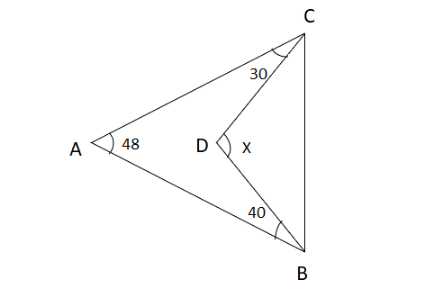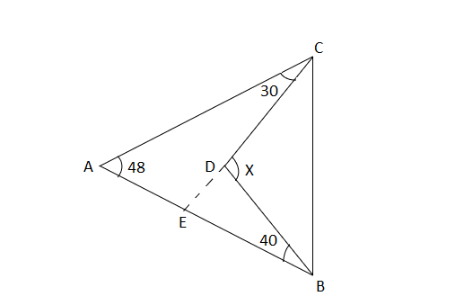QuestionAnswers

# Find the value of $x$ in the given figure given below:A. ${118^ \circ }$ B. ${20^ \circ }$ C. ${72^ \circ }$ D. ${223^ \circ }$Verified
92.1k+ views
Hint: In this question we have to find the value of $x$ . To find the value of $x$ we will use the sum of all interior angles of a triangle property and straight angle or straight-line definition. By using these properties, we can find the value of $x$ .
The sum of all interior angles of the triangle is ${180^ \circ }$ .
The angle of the straight line is ${180^ \circ }$ .

Consider the given figure. Draw a straight line from a point D which bisects the line $AB$ . Let name the point as E which bisects the line $AB$ .
Using the property “the sum of all interior angles of a triangle is ${180^ \circ }$ ”
Now consider the $\vartriangle ACE$
The sum of all interior angles is,
${48^ \circ } + {30^ \circ } + a = {180^ \circ }$
$\Rightarrow {78^ \circ } + a = {180^ \circ }$
$\Rightarrow a = {180^ \circ } - {78^ \circ }$
$\Rightarrow a = {102^ \circ }$
Therefore the $\left| \!{\underline {\, E \,}} \right.$ in the $\vartriangle ACE$ is ${102^ \circ }$ .Now consider the straight line $AB$ where E is the midpoint of $AB$ . We know that the angle of the straight line is ${180^ \circ }$ . We know value of the $\left| \!{\underline {\, {AEC} \,}} \right.$ is ${102^ \circ }$ .
Therefore, we have $\left| \!{\underline {\, E \,}} \right. = \left| \!{\underline {\, {AEC} \,}} \right. + \left| \!{\underline {\, {CEB} \,}} \right.$
$\Rightarrow \left| \!{\underline {\, E \,}} \right. = {102^ \circ } + y$
$\Rightarrow {180^ \circ } = {102^ \circ } + y \\ \Rightarrow y = {180^ \circ } - {102^ \circ } \;$
$\Rightarrow y = {78^ \circ }$
Now let us consider the $\vartriangle DEB$
The sum of all interior angles is,
${40^ \circ } + {78^ \circ } + z = {180^ \circ }$
$\Rightarrow {118^ \circ } + z = {180^ \circ }$
$\Rightarrow z = {180^ \circ } - {118^ \circ }$
$\Rightarrow z = {62^ \circ }$
Therefore $\left| \!{\underline {\, {EDB} \,}} \right.$ IS ${62^ \circ }$ .
Now consider the straight line $EC$ where D is the midpoint of $EC$ . We know that the angle of the straight line is ${180^ \circ }$ . We know value of the $\left| \!{\underline {\, {DEB} \,}} \right.$ is ${62^ \circ }$ .
Therefore, we have $\left| \!{\underline {\, D \,}} \right. = \left| \!{\underline {\, {EDB} \,}} \right. + \left| \!{\underline {\, {CDB} \,}} \right.$
$\Rightarrow \left| \!{\underline {\, D \,}} \right. = {62^ \circ } + b$
$\Rightarrow {180^ \circ } = {62^ \circ } + b \\ \Rightarrow b = {180^ \circ } - {62^ \circ } \;$
$\Rightarrow b = {118^ \circ }$
Therefore, the value of $x$ is ${118^ \circ }$ .
So, the correct answer is “Option A”.

Note: Candidates should be careful in determining the angles which are unknown. Here use these properties. The sum of all interior angles of the triangle is ${180^ \circ }$ and the angle of the straight line is ${180^ \circ }$ . Or we can use another property: the sum of interior angles is equal to the opposite exterior angle.